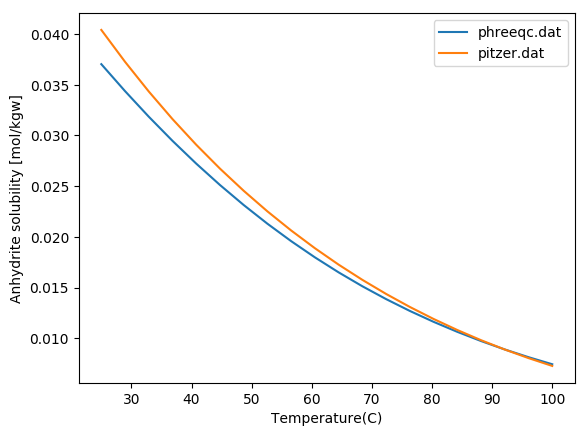# Calling Phreeqc from Julia: a tutorial

In this post, I'm going to show how we can call Phreeqc from Julia to calculate the solubility of minerals in a solution. The workflow is very useful specially when one wants to conduct a sensitivity analysis and plot the results. Here is my workflow:

• Create a Phreeqc input file in Julia
• Save it to a text file
• Call Phreeqc from Julia to run the created input file
• Read the results from the output file created by Phreeqc
• Plot the results

If you are on windows, you need to add phreeqc to the path so you can call it from the command line.

### create a Phreeqc simulation file¶

The following string is a phreeqc input file that mixes 10.0 moles of anhydrite in 1 kg of demineralized water at 50 degree Celsius and 250 atm, and writes the molality of Ca ion in the aqueous solution to an output file named anhydrite_solubility.csv:

SOLUTION 1 Pure water
pH      7.0
temp    50.0
pressure  10
EQUILIBRIUM_PHASES 1
Anhydrite       0.0     10.0
SELECTED_OUTPUT
-file   anhydrite_solubility.csv
-reset false
-totals     Ca
END

You can save this code in a file names e.g., anhydrite_sol.phr, and run it by

phreeqc anhydrite_sol.phr

if you have phreeqc installed and in your path. In windows, you can download phreeqc for windows and run it.

### Sensitivity analysis¶

My purpose here is to study the effect of thermodynamic models and databases, temperature, and pressure on the predicted solubility of anhydrite in water. It can be done manually, but I like to do it automatically from a Julia script. You ca do it in Matlab or any other tools of your choice.

### The workflow¶

We first create the Phreeqc input file as an string in Julia. Then, we save that script into a file and call phreeqc to run it. We save the results in an output file and read it in Julia. Finally we plot the results. The following script shows how this can be done in Julia. We need to use the packages PyPlot for visualization and DataFrames for reading the output file.

In :
using PyPlot, DataFrames

In :
T_min = 25.0     # degC minimum temperature
T_max = 100.0    # degC maximum temperature
p_atm = 250       # atm pressure
T_range = collect(linspace(T_min, T_max, 20))
data_base = ["phreeqc.dat", "pitzer.dat"]
for db in data_base
anhydrite_sol = zeros(0)
for T in T_range
phreeqc_input =
"""
DATABASE /usr/local/share/doc/phreeqc/database/$db SOLUTION 1 Pure water pH 7.0 temp$T
pressure  \$p_atm
EQUILIBRIUM_PHASES 1
Anhydrite       0.0     10.0
SELECTED_OUTPUT
-file   anhydrite_solubility.csv
-reset false
-totals     Ca
END
"""
# save it to a file
ph_file=open("phreeqc_input_file", "w")
write(ph_file, phreeqc_input)
close(ph_file)
# run it
run(phreeqc phreeqc_input_file)
# plot the results
push!(anhydrite_sol, ph_res[:Ca][end])
end
IJulia.clear_output()
plot(T_range, anhydrite_sol)
end
legend(data_base)
xlabel("Temperature(C)")
ylabel("Anhydrite solubility [mol/kgw]");You probably need to change the path to the Phreeqc databeses in line 11 of the above code. Play with the value of pressure and see what happens. It is truely fascinating that increasing pressure can affect the solubility that much. Don't forget that it can only be captured by the Pitzer model in Phreeqc.
There is another way of calling Phreeqc from Julia that I will discuss later.